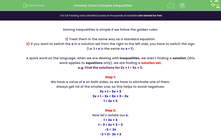# Solve Complex Inequalities

In this worksheet, students will practise solving multi-stage inequalities, where they may need to switch the sign at the end or may involve more than two elements.Key stage:  KS 4

Year:  GCSE

GCSE Subjects:   Maths

GCSE Boards:   AQA, Eduqas, OCR, Pearson Edexcel,

Curriculum topic:   Algebra

Curriculum subtopic:   Solving Equations and Inequalities Algebraic Inequalities

Difficulty level:#### Worksheet Overview

Solving inequalities is simple if we follow the golden rules:

1) Treat them in the same way as a standard equation.

2) If you want to switch the x in a solution set from the right to the left side, you have to switch the sign (i.e. 1 > x is the same as x > 1).

A quick word on the language, when we are dealing with inequalities, we aren't finding a solution (this word applies to equations only), we are finding a solution set.

e.g. Find the solutions for 2x + 1 < 5x + 3.

Step 1:

We have a value of x on both sides, so we have to eliminate one of them.

Always get rid of the smaller one, as this helps to avoid negatives.

3x + 1 < 5x + 3

3x + 1 - 3x < 5x + 3 - 3x

1 < 2x + 3

Step 2:

Now let's isolate our x:

1 < 2x + 3

1 - 3 < 2x + 3 - 3

-2 <  2x

-2 ÷ 2<  2x ÷ 2

-1 < x

Step 3:

Switch the inequality over if necessary:

x > -1

Now let's look at another example where our inequality has three parts:

Find the solutions for -5 ≤ 3x + 1 ≤ 7.

The key thing to remember is that we have to do the same to all parts of the inequality:

-5 ≤ 3x + 1 ≤ 7

-5 - 1 ≤ 3x + 1 - 1 ≤ 7 - 1

-6 ≤ 3x ≤ 6

-6 ÷ 3≤ 3x ÷ 3 ≤ 6 ÷ 3

-2 ≤ x ≤ 2

In this activity, you will find solutions for complex inequalities. You may need to switch the sign at the end of your working out may involve more than two elements. Our working for these questions can be long and complex, so make sure you have a pen and paper handy to record yours and then compare it to what our maths teacher has written as an example solution.

### What is EdPlace?

We're your National Curriculum aligned online education content provider helping each child succeed in English, maths and science from year 1 to GCSE. With an EdPlace account you’ll be able to track and measure progress, helping each child achieve their best. We build confidence and attainment by personalising each child’s learning at a level that suits them.

Get started